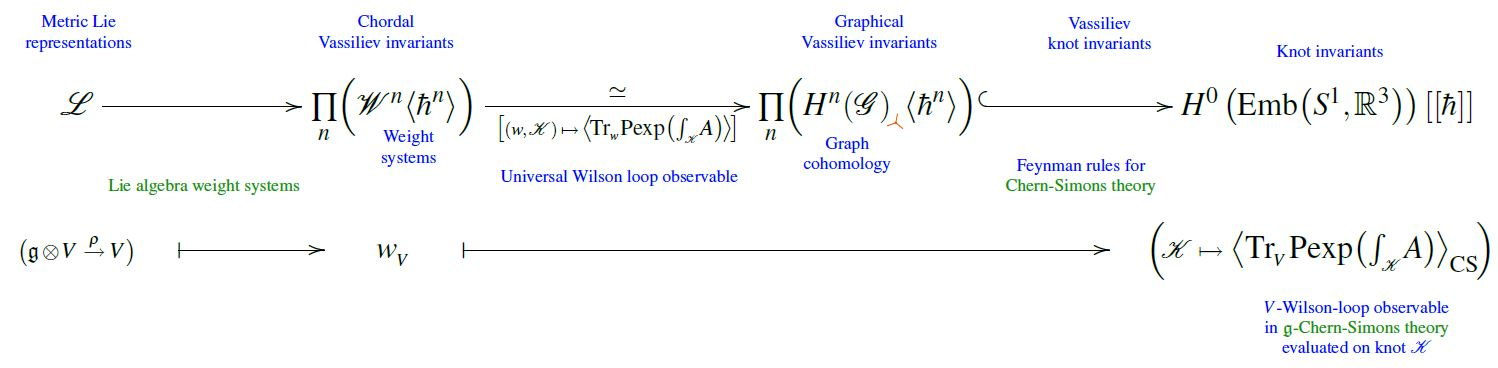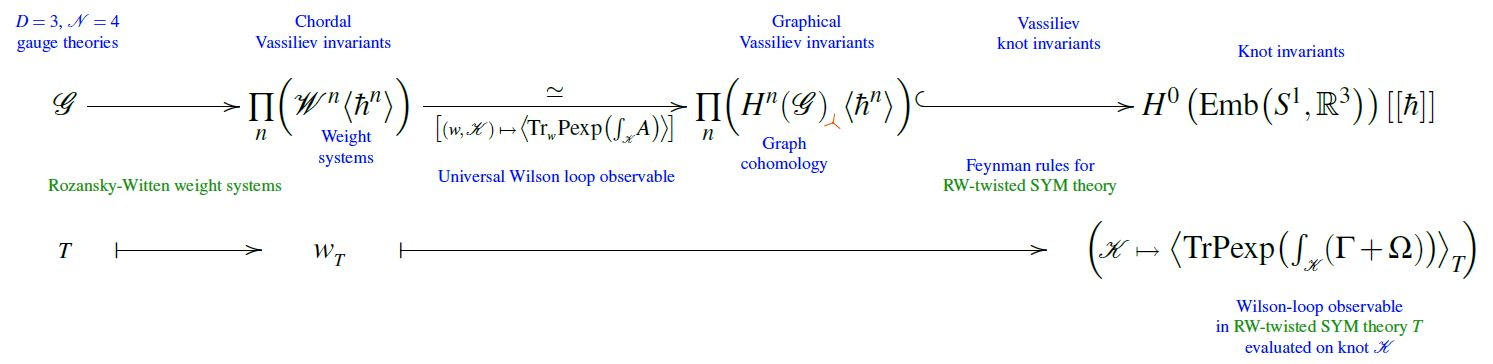# nLab Rozansky-Witten theory

Contents

### Context

#### Knot theory

knot theory

Examples/classes:

Types

knot invariants

Related concepts:

category: knot theory

# Contents

## Idea

What is called Rozansky-Witten theory (Rozansky-Witten 96) is a topological twist of D=3 N=4 super Yang-Mills theory, or else the assignment of topological invariant quantum observables which such a perturbative quantum field theory assigns to a given 3-manifold $\Sigma^3$, or more generally to a Wilson loop knot inside a 3-manifold – the Rozansky-Witten invariants.

The key result of Rozansky-Witten 96 is that, after gauge fixing and some subtle field identifications, the Feynman rules of RW-twisted D=3 N=4 super Yang-Mills theory are those of perturbative Chern-Simons theory, in that the only relevant propagator is the Chern-Simons propagator and the only relevant Feynman diagrams are trivalent, except that the Lie algebra weights assigned by Chern-Simons theory to a Feynman-Jacobi diagram, are replaced by other weight systems, now called Rozansky-Witten weight systems.

Precisely: Recalling that perturbative Chern-Simons Wilson loop observables are given by evaluating Lie algebra weight systems on the Jacobi diagram-valued universal Vassiliev invariantwe have that Wilson loop Rozansky-Witten invariants (Rozansky-Witten 96 (2.26)) are given by evaluating Rozansky-Witten invariants on the Jacobi diagram-valued universal Vassiliev invariantgraphics from Sati-Schreiber 19c

These Rozansky-Witten weight systems depend only on the hyperkähler manifold $\mathcal{M}^{4n}$ which is the (classical) Coulomb branch of the RW-twisted D=3 N=4 super Yang-Mills theory, and in fact they are independent of the Riemannian geometry of $\mathcal{M}^{4n}$ and depend only on the underlying holomorphic symplectic manifold (Kapranov 99). Generally, they are defined for $\mathcal{M}^{4n}$ any hyperkähler manifold which is either asymptotically flat (ALE spaces) or compact topological space (compact hyperkähler manifolds).

Via the equivalent reformulation by Kapranov 99 one finds (Roberts-Willerton 10) that the Rozansky-Witten invariants are structurally Lie algebra weight systems themselves, but internal to the derived category of coherent sheaves of $\mathcal{M}^{4n}$ and composed with an integration over $\mathcal{M}^{4n}$ which makes the resulting Dolbeault cohomology-valued weights become ground field-valued.

Konsevich 97 gives a formulation of Rozansky-Witten invariants via characteristic classes of foliations and Gelfand-Fuks cohomology. He devised a formal construction, again depending on a trivalent graph of a cohomology class of the Lie algebra of formal (in the sense of formal power series) Hamiltonian vector fields on any arbitrary finite-dimensional symplectic vector space. Characteristic classes of foliations may induce examples where this construction applies; one of the examples yields Rozansky-Witten .

## Properties

### As KK-compactification of M5-brane worldvolume

Rozansky-Witten theory may be identified with topologically twisted KK-compactification of the D=6 N=(2,0) SCFT on the M5-brane (Gukov-Putrov-Vafa 17, Sections 3.2 and 4.2)

## Examples

### Rozanzky-Witten Wilson loop of unknot is $\hat A$-genus

###### Proposition

(Rozansky-Witten Wilson loop of unknot is square root of A-hat genus)

For $\mathcal{M}^{4n}$ a hyperkähler manifold (or just a holomorphic symplectic manifold) the Rozansky-Witten invariant Wilson loop observable associated with the unknot in the 3-sphere is the square root $\sqrt{{\widehat A}(\mathcal{M}^{4n})}$ of the A-hat genus of $\mathcal{M}^{4n}$.

This is Roberts-Willerton 10, Lemma 8.6, using the Wheels theorem and the Hitchin-Sawon theorem.

Original articles:

Unified description of Rozansky-Witten weight systems as Lie algebra weight systems for Lie algebra objects in the derived category of quasi-coherent sheaves, and unified Wheels theorem:

Review:

Further relation to Chern-Simons theory:

• Sergei Gukov, Pavel Putrov, Cumrun Vafa, Sections 3.2 and 3.4 of: Fivebranes and 3-manifold homology, J. High Energ. Phys. (2017) 2017: 71 (arXiv:1602.05302)

• Cyril Closset, Heeyeon Kim, Section 6.1 of: Comments on twisted indices in 3d supersymmetric gauge theories, JHEP 08 (2016) 059 (arXiv:1605.06531)

Discussion of Rozansky-Witten theory as a boundary field theory:

Relation to equivariant cohomology:

• Sergei Gukov, Po-Shen Hsin, Hiraku Nakajima, Sunghyuk Park, Du Pei, Nikita Sopenko, Rozansky-Witten geometry of Coulomb branches and logarithmic knot invariants (arXiv:2005.05347)

• Jian Qiu, Rozansky-Witten theory, Localised then Tilted (arXiv:2011.05375)